# Electromagnetism Equations Explained

## Revision Notes on Electromagnetism

1) The electromagnetic force experienced by a current I placed in a magnetic field B.
The magnitude of this force is given by F= BIL sinθ, where L is the length of the conductor placed inside the field and θ is the angle between I and B.
The direction of the force is given by Fleming’s Left Hand Rule.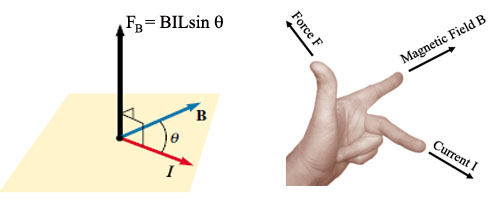2) Force on a charge moving into a uniform perpendicular magnetic field is given by F = BQv. Here the electromagnetic force provides the centripetal force for circular motion.

 r = mv/BQ.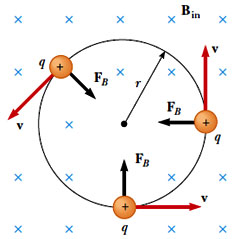3) Velocity Selector: In many experiments involving moving charged particles, it is important that the
particles all move with essentially the same velocity. This can be achieved by applying
a pair of crossed E and B fields oriented as shown below.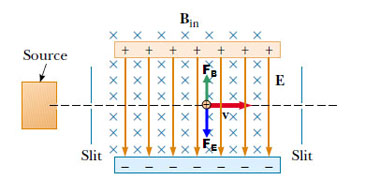The velocity of the charged particles that pass straight through the slits is given by equating the magnetic and electric forces

Bqv = qE and so v = E/B.

4) Magnetic flux density is defined as the force acting per unit current per unit length of the conductor when the conductor is placed at right angles to the field.

5) One tesla is the magnetic flux density of a field in which a force of 1 N acts on a conductor 1 m long which is carrying a current of 1 A and is perpendicular to the field.

6) Magnetic fields of current-carrying conductors

(a) Long straight wire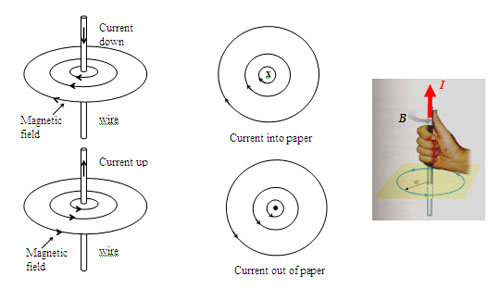Use the Right Hand Grip Rule to find the direction of the magnetic field.

(b) Flat circular coil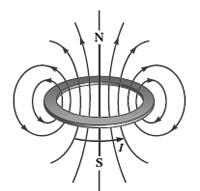(c) Solenoid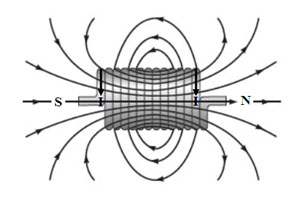For a long solenoid, the magnetic flux density inside the solenoid is approximately uniform, i.e. the B field lines are parallel to the axis of the solenoid.

7) Force between two parallel current carrying conductors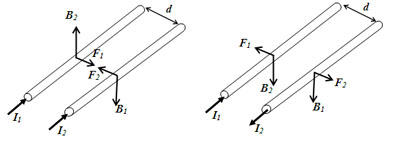Like currents attract, unlike currents repel.